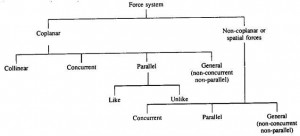# Which are the Different System of Force and Characteristic in Engineering Mechanics

Force is main criteria that you are going to deal with your designing of structures.There is so many force system you need to know for solving your problems in designing of structures.There are several important terms and definition you need to know in engineering mechanics.

Force can be defined as any action that tends to change the state of rest or uniform motion of a body.According to Newton’s first law of motion, you know that there should some external force required to accelerate a motion or move a particle from its rest position.

Unless no external force, the rigid body will move only at a constant velocity during its motion or rigid body does not move from its rest position.

## Characteristics of force system

The different characteristics of force systems are

1. The magnitude of the force
2. Point of application of the force
3. Direction
4. Line of action

## Different force system in mechanics

Force system is the set forces acting on a body or a group of bodies.Force system is classified according to the orientation of the lines or effect of the action of these force.

The system of force is mainly classified in Coplanar and Non-coplanar.### Coplanar forces and Non-coplanar forces

In coplanar forces, the line of action of all forces is lying on the same plane.If the lines of action of all the forces are lying on a different plane, then they are called as non-coplanar forces.

### Collinear forces

In a collinear force system, the line of action of the entire force system is in the same direction.

### Concurrent and Non-concurrent types of force system

If the line of line of action of all forces passing through a single point then the force system is called as a concurrent force system.Similarly, if the line of action of all forces does not pass through a single point, then they are called as non-concurrent forces.### Parallel system of forces

In a parallel system of forces, the line of actions of all forces is parallel to each other.The parallel system is again classified into like and unlike.If the forces are in same direction then it is like parallel system and if the forces are in opposite direction then it is unlike parallel system.

Hope you can understand the different system of force used in engineering mechanics to solve problems.If you like this post, don’t forget to share it.